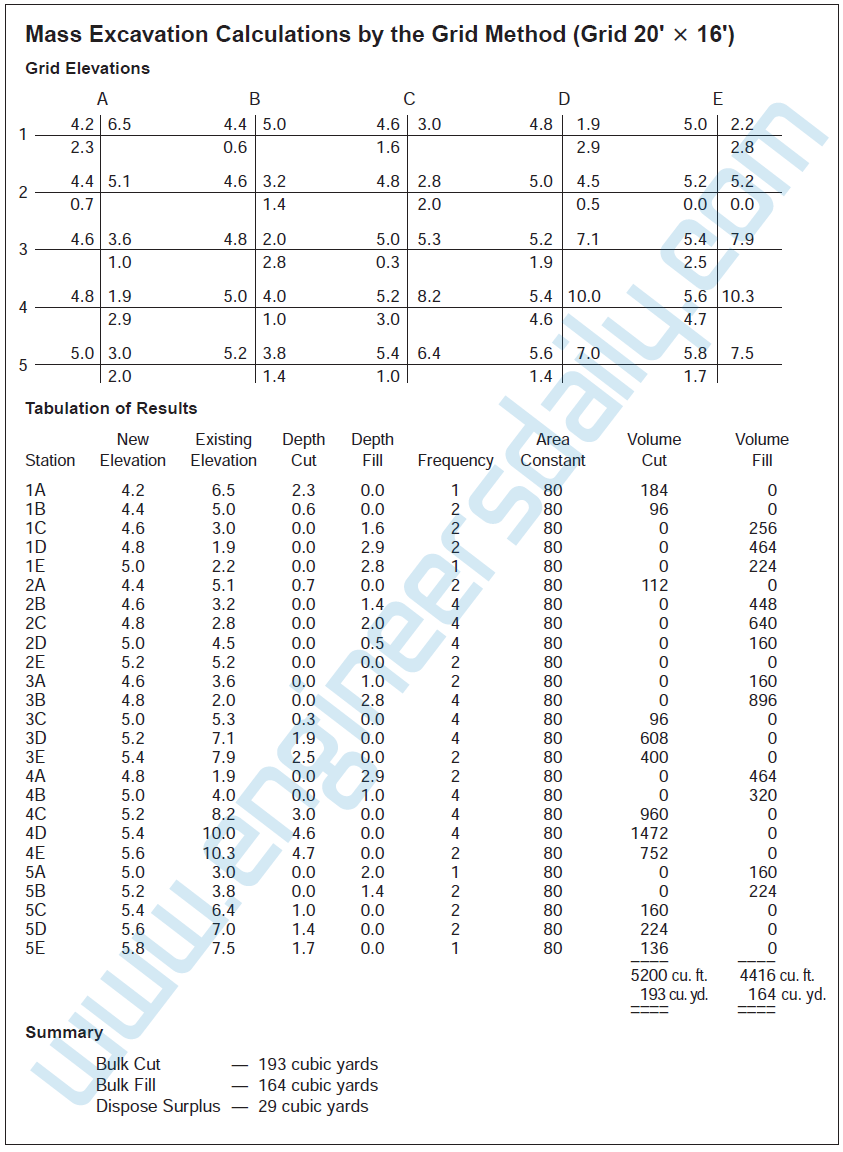# Calculation of Cut and Fill Using the “Grid Method”

Certainly the fastest, and probably the most accurate, way to calculate volumes of cut and fill over a site, when true scale drawings are available, is to use an electronic digitizer in conjunction with a software program specifically for this type of application. Here we consider an alternative “manual” method of obtaining the quantities of cut and fill called the “grid method.

Calculation by the “grid method” requires a survey of the site showing the elevation of the existing grade at each intersection point on the grid. The elevation of the required new grade is also plotted at each intersection point, and from these two elevations the depth of cut or fill can then be obtained at each point.
From here on, cut calculations are separate from fill calculations. To figure the volume of cut at an intersection point, the depth of cut at this point is multiplied by the area “covered” by that intersection point. Then, adding together all the individual cut volumes computed in this way will give the total volume of cut on the site. Following the same process using the fill depths will establish the individual and total fill volumes for the site. The area “covered” by an intersection point means the area that point applies to, as shown in Figure 1.

The accuracy of this method of calculating cut and fill volumes depends on the grid spacing; generally, the closer the grid spacing, the more accurate the results are.

Detailed Discussion

The use of the “grid method” to calculate volumes of cut and fill requires the estimator to consider the depth of cut or fill at each point where the grid lines intersect (station) on the survey grid and then determine the “area covered” by that station.

At each station on the grid, the elevation of the existing grade obtained from the site survey is noted in the top right quadrant. The elevation of the required new grade is noted in the top left quadrant. The difference between these grades gives the depth of fill or cut at this location. If it is a fill, the depth is noted in the bottom right quadrant; if a cut, the depth is noted in the bottom left quadrant.

Consider the accompanying survey grid. If the areas formed by the grid lines are “A” square feet, the “area covered” by point 1-A is one quarter of area “A,” but point 1-B applies to two areas so the “area covered” by this point is two quarters of area “A,” and so on as shown. The number of quarters of area “A” that the station point applies to is labeled the “frequency” when tabulating this data.Figure 1: Mass Excavation Calculations by Grid Method
However, a closer grid spacing leads to more calculations and a longer processing time. As this process is very repetitive, the processing time can be much reduced by using a computer program to perform the calculations. Please see Figure 2 for a complete calculation of volumes of cut and fill over a site using this “grid method” with a computer spreadsheet program.Figure 2: Sample Calculation Using Computer Spreadsheet Program
The method presented in this article can also be performed very effectively in MS Excel program. Thank you